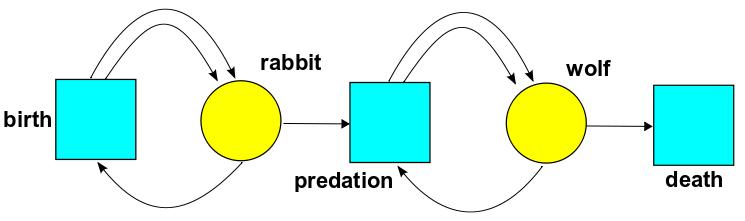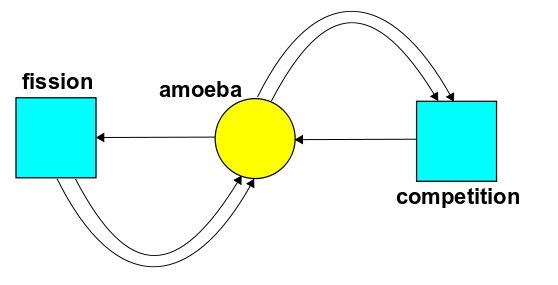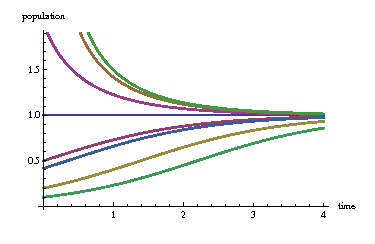# The Azimuth Project Quantum techniques for stochastic mechanics (course) lecture 2

## Quantum Techniques for Stochastic Mechanics

• Lecture 2 of 4

• Quantum Techniques for Stochastic Mechanics, by Jacob Biamonte, QIC 890/891 Selected Advanced Topics in Quantum Information, The University of Waterloo, Waterloo Ontario, Canada, (Spring term 2012).

• Given Aug 9th, 2012 in Waterloo Canada

### Lecture Content

• Review of lecture 1

• Probabilities vs amplitudes (chapter 4)

• Operators (creation and annihilation)

• Amoeba field theory

• Preamble to stochastic mechanics vs quantum mechanics

• Note: the lecture content here is to be read along with the youtube video of the course. It should contain what was written on the board. Without the video, it might not make sense.

### The master equation vs the rate equation

A stochastic petri net has

• $k$ species
• $x_i$ is the concetration of the ith species

we want to know

$\frac{d}{d t} x_i = ???$

one for each $1\leq i \leq k$.

• The RHS: sum of terms, one term for each transition in the Petri net

For each transition we end up with a term like this

$\frac{d}{d t} x_i = r (n_i - m_i)x_1^{m_1}\cdots x_k^{m_k}$
• $n_i$ is the number of times species $x_i$ appears in the output
• likewise for $m_i$ but instead for input

We will use index free notation.

$x = (x_1,\ldots, x_k) \in [0, \infinity)$

is the concentration vector and

• input vector: $m = (m_1, \ldots, m_k) \in N^k$

• output vector: $n = (n_1, \ldots, n_k) \in N^k$

• notation: $x^m = x_1^{m_1}\cdots x_k^{m_k}$

then

$\frac{d}{d t} = r(n - m)x^m$
• $T$ is the set of transitions $\tau \in T$
• $r(\tau)$ is the rate constant
$\frac{d}{d t} x = \sum_{\tau \in T} r(\tau) [n(\tau) - m(\tau)]x^m(\tau)$

Example• In birth, one rabbit comes in and two go out. This is a caricature of reality: these bunnies reproduce asexually, splitting in two like amoebas.

• In predation, one wolf and one rabbit come in and two wolves go out. This is a caricature of how predators need to eat prey to reproduce.

• In death, one wolf comes in and nothing goes out. Note that we are pretending rabbits don’t die unless they’re eaten by wolves.

• $R(t)$ rabbits

• $W(t)$ wolves

The rate equations of motion are

$\frac{d}{d t} R(t) = \beta (2-1)R(t) + \gamma (0-1) R(t) W(t)$
$\frac{d} {d t} W(t) = \delta (0-1) W(t) + \gamma (2-1) R(t) W(t)$

### The master equation

• Let $\psi_{n_1, \ldots, n_k}$ be the probability that we have $n_1$ of the first thing, $n_2$ of the second, etc.

• The master equation says how these “things” change with time

• It contains all possible “histories” of the possible interactions

Let $n = (n_1, \ldots, n_k) \in N^k$ and $\psi_n = \psi_{n_1}, \ldots, \psi_{n_k}$ and then write a monomial

$z^n = z^{n_1}_1\cdots z^{n_k}_k$

and express any stochastic state as

$\Psi = \sum_{n\in N^k}\psi_n z^n$

A state has

$\Psi |_{z=1} = \sum_n\psi_n = 1$
• The simplest example is a monomial $z^{n_1}_1\cdots z^{n_k}_k$ where we are 100 percent sure that we have $n_1$ of the first species, etc. We call this a pure state.

The master equation provides a description of how $\Psi$ changes with time.

$\frac{d}{d t} \psi(t) = H \psi(t)$
• $H$ will be built from creation and annihilation operators

• (annihilation) $a_i \psi = \frac{d}{d z_i} \psi$

• (creation) $a_i^\dagger \psi = z_i \psi$

• $r(\tau)$ rate constant for transition $\tau\in T$

• $n(\tau)$ output vector

• $m(\tau)$ input vector

then

$H = \sum_{\tau\in T} r(\tau) [ a^{\dagger n(\tau)} - a^{\dagger m(\tau)}]a^{m(\tau)}$

with

$a^{m(\tau)} = a_1^{m_1(\tau)}\cdots a_k^{m_k(\tau)}$ $a^{\dagger m(\tau)} = a_1^{\dagger m_1(\tau)}\cdots a_k^{\dagger m_k(\tau)}$

How can we understand each term?

• The first term $a^{\dagger n(\tau)} a^{m(\tau)}$ describe how $m_i(\tau)$ things of the ith species get annihilated and $n_i(\tau)$ things of the ith get created

• The second term $a^{\dagger m(\tau)} a^{m(\tau)}$ says how the probability that nothing happens goes down as time passes.

Consider againhere

$\psi = \sum_n \psi_n z^n$

where

$z^n = z_1^{n_1} z_2^{n_2}$
• $n_1$ rabbits
• $n_2$ wolves

and so

$\psi_n = \psi_{n_1, n_2}$

is the probability of having $n_1$ rabbits and $n_2$ wolves. These probabilities evolve according to

$\frac{d}{d t} \psi(t) = H \psi(t)$
$H = \beta B + \gamma C + \delta D$
$H = \sum_{\tau\in T} r(\tau) [ a^{\dagger n(\tau)} - a^{\dagger m(\tau)}]a^{m(\tau)}$

order the input and output vectors as $(R, W)$ then

• $m(\tau_1) = (1,0)$

• $m(\tau_2) = (1,1)$

• $m(\tau_3) = (0,1)$

• $n(\tau_1) = (2,0)$

• $n(\tau_2) = (0,2)$

• $n(\tau_3) = (0,0)$

$B = r_1[ a_1^\dagger a_1^\dagger - a_1^\dagger] a_1$
$C = r_2[ a_2^\dagger a_2^\dagger - a_1^\dagger a_2^\dagger]a_1a_2$
$D = r_3[1-a_2^\dagger]a_2$

### Basic properties of stochastic mechanics

In the lecture, we briefly recalled some of the basic properties of stochastic mechanics, which can be found in the book, as well as several of the blog articles on azimuth.

### Amoeba field theoryIt shows a world with one state, amoeba with concentration $A(t)$, and two transitions:

reproduction, where one amoeba turns into two. Let’s call the rate constant for this transition $\alpha$.

competition, where two amoebas battle for resources and only one survives. Let’s call the rate constant for this transition $\beta$.

Here

$\frac{d}{d t} A(t) = \alpha A - \beta A^2$

which has solutionsequilibrium. The horizontal blue line corresponds to the case where the initial population $P_0$ exactly equals the carrying capacity. In this case the population is constant.

dieoff. The three decaying curves above the horizontal blue line correspond to cases where initial population is higher than the carrying capacity. The population dies off over time and approaches the carrying capacity.

growth. The four increasing curves below the horizontal blue line represent cases where the initial population is lower than the carrying capacity. Now the population grows over time and approaches the carrying capacity.

#### Quick intro to the deficiency zero theorem

The deficiency zero theorem gives conditions on the network that allow one to state that the master equation has a unique equilibrium solution.

For a harmonic oscillator,

$\Delta p \Delta q \geq \hbar/2$

A coherence state of the harmonic oscillator minimizes $\Delta p \Delta q$ with $\Delta p = \Delta q$

We can write down

$\Psi = \sum_{n=0}^{\infinity} \psi_n z^n$
$\Psi = e^{c z} = \sum_{n=0}^{\infinity} \frac{c^n}{n!}z^n$

For amoebas

$\Psi = \frac{e^{c z}}{e^c} = e^{-c} \sum_{n=0}^{\infinity} \frac{c^n}{n!}z^n$

now the probability distribution $\psi_n = e^{-c} \frac{c^n}{n!}$ is called a poisson distribution. The expected number of amoebas is

$\sum N \Psi = \sum a^\dagger a \Psi = \sum a^\dagger a \frac{e^{c z}}{e^c}$
$= \sum z \frac{d}{d z} \frac{e^{c z}}{e^c} = c\sum z \frac{e^{c z}}{e^c} = c \sum a^\dagger \Psi = c \sum \Psi$